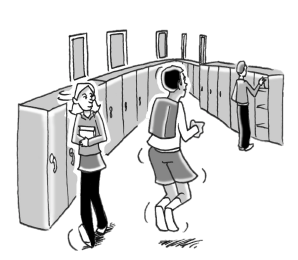### Home > CC3 > Chapter 7 > Lesson 7.3.2 > Problem7-104

7-104.

When Malcolm hops $10$ times down the hallway, he travels $12$ feet. How many times would he need to hop to travel to his class, $90$ feet away?

Set up a proportion.

$\frac{10\ \text{hops}}{12\ \text{feet}}=\frac{x\ \text{hops}}{90\ \text{feet}}$

$900=12x$
Divide both sides by $12$ to figure out how many times Malcom would need to hop to travel to class.